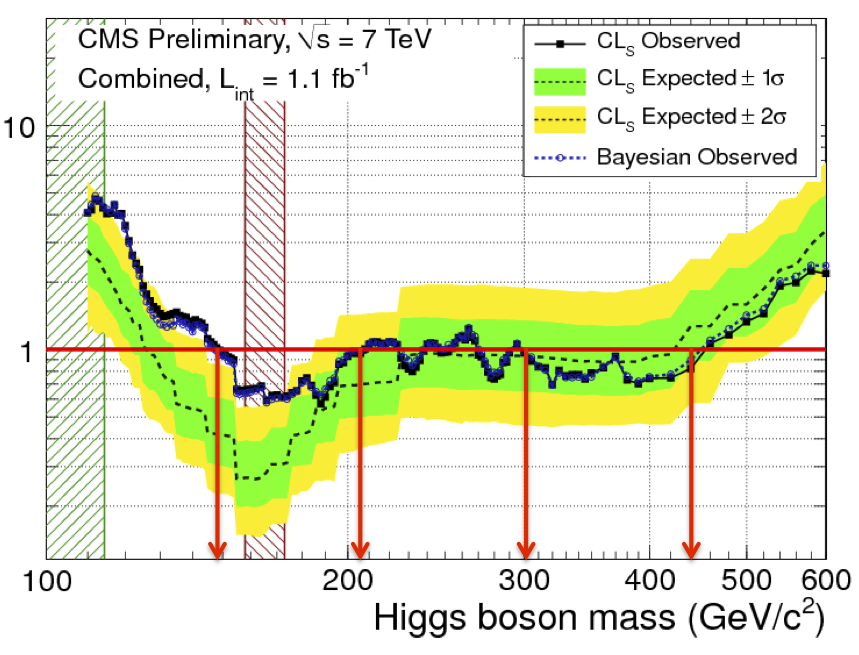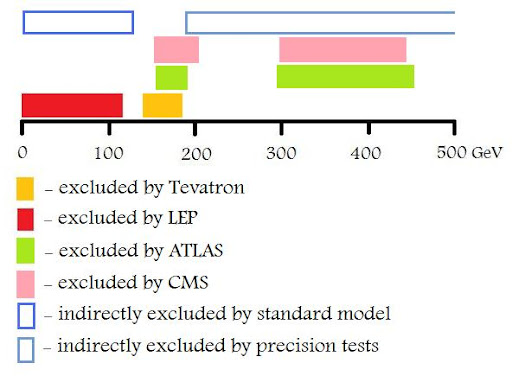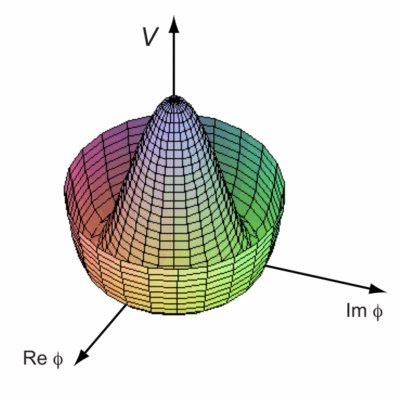### A circle around Higgs boson

After the post about D0 abstracts, I return to write about Higgs boson after the last Fermilab's press release about the mass limit of Higgs boson. Combinig data from D0 and CDF, Tevatron's limits are 114-137 GeV/c2. The results was presented last week in Grenoble at the EPS High-Energy Physics conference, that it will finish on the 27th July.
During the same conference also LHC's experiments presented their first results, analyzed in about one month! And the conclusion seems un-huppy for Tevatron: the Fermilab's particle accelerator has only one chance to find Higgs boson before LHC. Why? We can simply see the following plots presented by ATLAS and CMS (via Résonaances, Tommaso Dorigo):The two european experiments presented only a little region around 115 GeV/c2, the Tevatron's region, to 140 GeV/c2. The data from this region are probably analized and published before the end of the year, so we must wait only some months to know if Tevatron could found Higgs or not(1).
Tomasso examined in details some CMS preprint in which they are studied a lot of Higgs production channels, and also Philip Gibbs write a great summary about LHC presentations, who realize a great conclusion plot:But, what is Higgs boson?
In a series of paper François Englert and Robert Brout(2), Peter Higgs(3) and Gerald Guralnik, C. R. Hagen, and Tom Kibble(4) proposed a broken symmetry mechanism well known as Englert-Brout-Higgs-Guralnik-Hagen-Kibble mechanism. The first application of the mechanism is dued by Weinberg and Salam to the electroweak symmetry breaking and it can explain a lot of observations, like the ratio between W and Z bosons masses (more details at LEP, the collider who confirmed a lot of consequences of Higgs mechanism), but the first mention of the existense of a massive field, a boson, is dued by Peter Higgs, and this is the reason of the new boson's name.
The boson's emergence is directly consequence of the spontaneous symmetry breaking. For example we have a spin system represented by the following hamiltonian:
$H = -\frac{1}{2} \sum_{i,j} J_{ij} S_i S_j$
where $S_i$ is the spin of $i$-th site, and $J_{ij}$ is the strenght of the interaction that depends only by the distance between $i$ and $j$.
This model is invariant under rotation, unless $J$ is positive: in this case the spins' directions are arbitrary, and the symmetry is broken!
There are also others models that present a symmetry breaking. For example the Goldstone's relativistic lagrangian:
$L \partial_\mu \phi^* \partial^\mu \phi - V(\phi)$
where $\phi$ is a complex scalar field, and
$V(\phi) = m^2 \phi^* \phi + \frac{1}{2} \lambda (\phi^* \phi)^2$
where $m$ and $\lambda$ are mass and self-interaction coupling constant of the field respectively.
In this case, if $m^2 > 0$, there is an invariant under a global change of phase
$\phi (x) \rightarrow \phi (x) e^{i \alpha}$
but for $m^2 < 0$ there is an unstable equilibrium and the potential assumed the following form:(ScholarPedia)
and so the system present a symmetry breaking.
Another lagrangian in which there is a similar mechanism is
$L = D_\mu \phi^* D^\mu \phi - \frac{1}{4} F_{\mu \nu} F^{\mu \nu} - V(\phi)$
where
$D_\mu \phi = \partial_\mu + i e A_\mu \phi$
$F_{\mu \nu} = \partial_\mu A_\nu - \partial_\nu A_\mu$
In this case the lagrangian is invariant unbder the following gauge transformation:
$\phi (x) \rightarrow \phi (x) e^{i \alpha (x)}$
$A_\mu (x) \rightarrow A_\mu (x) - \frac{1}{e} \partial_\mu \alpha (x)$
And at the end of calculation it is emerged, caused by symmetry breaking, a massive field:
$B_\mu = A_\mu + \frac{1}{ev} \partial_\mu \varphi_2$
whit $ev$ is the field's mass.
In Englert-Brout-Higgs-Guralnik-Hagen-Kibble mechanism a massive field emerges from a non-abelian lagrangian and this field is associated to a boson, the Higgs boson.

Conclusion: Higgs boson is the particle responsible for the mechanism that makes the other elementary particles gain mass. It is introduced in Standard Model in order to correct the unconsistences of the model, like the presence of processes with probability greater than 1. To some theorists the introduction of Higgs boson is a mathematical stratagem, and the corrections to SM could be done with a Higgsless model, and at the other hand the Higgs mechanism has made a series of previsions some of them verified by LEP.
In every case (Higgs exists or not) in this moment we can only obtain the limits of his existence: in a little region between 115 Gev and 145 GeV about, and a region between 200 GeV to 300 GeV about. The first region present a very little signal (it's modest also for CMS), so an absence of a signal in this region sign the end of Higgs mechanism (I think that SM, in this case, has more possibility to complete itself with alternatives), but the discover of a massive boson in the second, great region, that it is excluded by Standard Model, it signs the death of the more succesful theory in very great energy. And probably this is the right answer.

Thanks to Salvatore Fazio, who shares via e-mail the first news about seminars, and to Marco Delmastro for the little summary of the Higgs day (in italian: part 1 and part 2).
In conclusion, you can see a little Storify about the recently news about Higgs.

(1) Tommaso and Jester are a bit skeptic about the possibility to found Higgs in Tevatron's limits, but at the other hand Philip is more optimistic.
(2) Broken Symmetry and the Mass of Gauge Vector Mesons, (1964)
(3) Broken Symmetries and the Masses of Gauge Bosons, (1964)
(4)
Global Conservation Laws and Massless Particles, (1964)

Kibble, T., & Rajantie, A. (2009). Englert-Brout-Higgs-Guralnik-Hagen-Kibble mechanism Scholarpedia, 4 (1) DOI: 10.4249/scholarpedia.6441